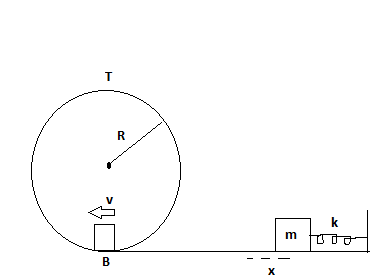# A block of 0.5 kg is pushed against a horizontal spring of negligible mass, compressing the...

## Question:

A block of 0.5 kg is pushed against a horizontal spring of negligible mass, compressing the spring a distance of x as shown in in figure. The spring constant is 450 n/m. When released the block travels along a frictionless horizontal surface to point B, the bottom of the vertical circular track of radius R = 1 m and continues to move up the track. The circular track is not smooth. The speed of the block at the bottom of the vertical circular track is v = 12 m/s, and the block experiences an average frictional force of 7.0 N while sliding up the curved track.

A) What was the initial compression, x of the spring?

B) What is the speed of the block at the top of the circular track?

C) Does the block reach the top of the track, or does it fall off before reaching the top?## Conservation of energy:

If the external forces applied to the system are conservative forces, then the entire mechanical energy of the system remains conserved. A ball rotating over a rough surface will not follow the law of conservation as some amount of energy is going in the surrounding in the form of heat.

Given data

• Mass of the block is {eq}m = 0.5\;{\rm{kg}} {/eq}
• Spring constant is {eq}k = 450\;{\rm{N/m}} {/eq}
• Radius of the circular track is {eq}R = 1\;{\rm{m}} {/eq}
• Speed of the block is {eq}v = 12\;{\rm{m/s}} {/eq}
• Frictional force is {eq}{F_f} = 7\;{\rm{N}} {/eq}

The expression for the conservation of energy is

{eq}\dfrac{1}{2}k{x^2} = \dfrac{1}{2}m{v^2} {/eq}

The expression for the work done is

{eq}W = mgh {/eq}

Substituting the values for the compression in the spring

{eq}\begin{align*} \dfrac{1}{2}\left( {450} \right){x^2} &= \dfrac{1}{2}\left( {0.5} \right){\left( {12} \right)^2}\\ x &= 0.4\;{\rm{m}} \end{align*} {/eq}

Substituting the values for the work done by the gravity

{eq}\begin{align*} W &= 0.5 \times 9.81 \times \left( {2 \times 1} \right)\\ W &= 9.81\;{\rm{J}} \end{align*} {/eq}

The work done by the friction force can be calculated as

{eq}\begin{align*} {W_f} &= 7 \times \pi \times 1\\ {W_f} &= 22\;{\rm{J}} \end{align*} {/eq}

The net work done is {eq}22 + 9.81 = 31.81\;{\rm{J}} {/eq}

Substituting the values for the kinetic energy

{eq}\begin{align*} KE &= \dfrac{1}{2}\left( {0.5} \right){\left( {12} \right)^2}\\ KE &= 36\;{\rm{J}} \end{align*} {/eq}

Since the kinetic energy is more, the block reach the top of the track.

By applying net work energy theorem,

The final kinetic energy can be calculated as

{eq}\begin{align*} K{E_f} &= 36 - 31.81\\ \dfrac{1}{2}\left( {0.5} \right){v_f}^2 &= 5.81\\ {v_f} &= 4.09\;{\rm{m/s}} \end{align*} {/eq}

Therefore, the final velocity of the block at the top is {eq}4.09\;{\rm{m/s}}.{/eq}